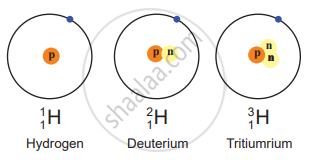# Answer the following question. Name the isotopes of hydrogen. Write their atomic composition schematically and explain which of these is radioactive? - Chemistry

Name the isotopes of hydrogen. Write their atomic composition schematically and explain which of these is radioactive?

#### Solution

1. Hydrogen has three isotopes i.e., hydrogen $\ce{(_1^1H)}$, deuterium $\ce{(_1^2H)}$ and tritium $\ce{(_1^3H)}$ with mass numbers 1, 2 and 3 respectively.
2. They all contain one proton and one electron but different number of neutrons in the nucleus.
3. Atomic composition of isotopes of hydrogen:
 Name of the isotope Symbol Atomic number Z Atomic mass number A Neutron number N Abundance Stability Hydrogen $\ce{H or _1^1H or H-1}$ 1 1 0 99.98% Stable Deuterium $\ce{D or _1^2H or H-2}$ 1 2 1 0.015% Stable Tritium $\ce{T or _1^3H or H-3}$ 1 3 2 Trace Radioactive
4. Tritium is a radioactive nuclide with a half-life period of 12.4 years and emits low energy β particles.
5. Schematic representation of isotopes of hydrogen is as follows:Concept: Hydrogen
Is there an error in this question or solution?

#### APPEARS IN

Balbharati Chemistry 11th Standard Maharashtra State Board
Chapter 8 Elements of Group 1 and 2
Exercises | Q 3. (D) | Page 122